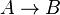# Difference between revisions of "Number of explainers and learners"

This page classifies learning situations by the number of explainers (e.g. teachers) and number of learners (e.g. students) present. Each learning situation can be visualized as a directed graph where an arrow from$A$ to$B$ ($A \to B$) means "$A$ explains to$B$".